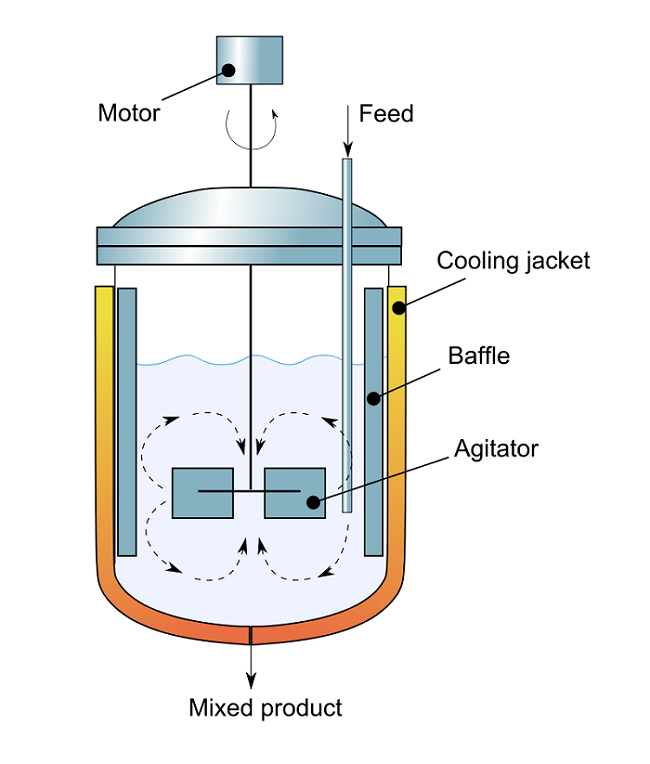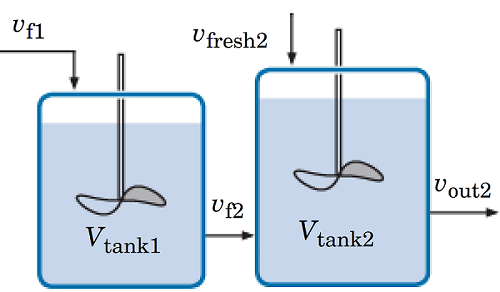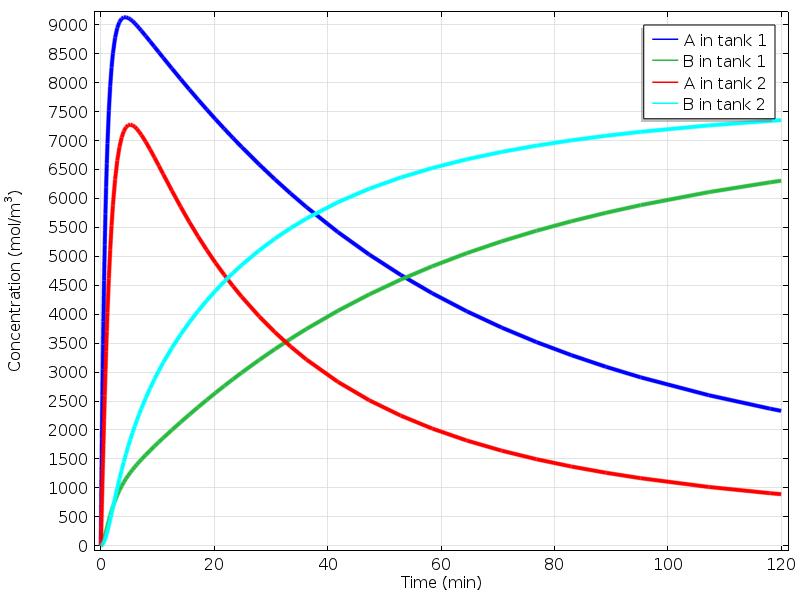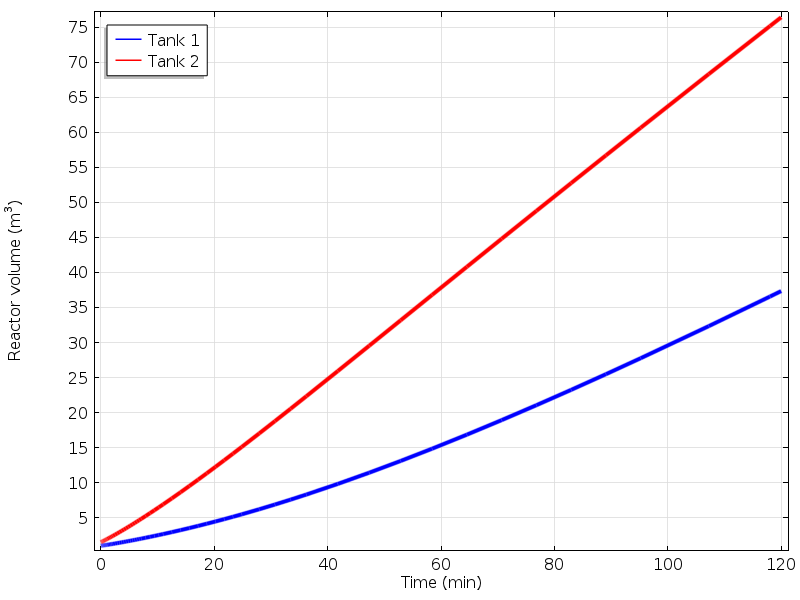# Simulating an Ideal Stirred Tank Reactor System

February 24, 2015

Continuous stirred tank reactors (CSTRs), or ideal stirred tank reactors, are frequently used in the chemical and biochemical industries. This reactor type operates at steady state and because of its good mixing properties, it is assumed that the composition throughout the reactor is uniform. Using a new model in the Reaction Engineering interface, we can visualize the dynamics within an ideal system of tank reactors.

### The Application of Ideal Stirred Tank Reactors

In chemical engineering, reactors that feature well-mixed conditions and liquid level control are quite common. Within such ideal stirred tank reactors, perfect mixing is assumed and the output composition is the same as the composition of the material within the reactor. In such systems, reactants are constantly introduced into the reactor and products are continuously removed. The figure below illustrates the different components of a continuous stirred tank reactor.A schematic of a continuous flow stirred tank reactor. (“Cross-sectional diagram of Continuous flow stirred-tank reactor” by Aushulz — Own work. Licensed under Creative Commons Attribution Share-Alike 3.0, via Wikimedia Commons).

### Modeling an Ideal System of Tank Reactors in Series

Available in COMSOL Multiphysics version 5.0, the Ideal Stirred Tank Reactor System model uses the new reactor type called Generic CSTR, a recent addition to the Reaction Engineering interface (learn about this and additional updates to the interface here).

This model is designed to solve for a liquid-phase, first-order irreversible reaction in which reactant A forms product B. The reaction occurs in a system of two ideal reactors in series. The figure below shows the reactors. The first reactor has a volume of vtank1 = 1 m3 and the second reactor has a volume of vtank2 = 1.5 m3 initially.A detailed overview of the reactor system.

At the beginning, the reactors are both charged with just solvent. vf1 = 1 m3/min is the volumetric flow rate of A with solvent fed to the first tank. The rate at the outlet of the first reactor is set to vout1 = 0.9m3/min. This outlet stream is fed to the second tank, vf2 = vout1. A fresh supply of A with solvent is also fed to the second tank at a rate of vfresh2 = 0.5 m3/min. The outlet flow rate from the second tank is regulated to vout2 = 1 m3/min.

Within this model, two stop conditions have been implemented. The computations will stop if any of the reactor volumes is 1% or less of the initial volume.

The first figure below highlights the concentrations of species A and B in each tank.Comparing concentrations in the two tanks.

The next graph illustrates the variation of the volumes in each of the tanks. From these volumes — and with known cross-sectional areas of each reactor — the liquid levels can be computed.The volume of the reactors.